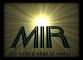# What is Y'CBCR?

The little discussion here is an incredible simplification of really neat but very complex topic which involves 50 years of broadcast video engineering and a 100 years of study of the human visual system. If you want anything even resembling the full story, you should check out the references at the end.

Y'CBCR denotes a colorspace, that is, a particular way of specifying the pixel values in a color image. Y'CBCR is the standard colorspace used for digital video processing (as defined by ITU-R BT.601, formerly known as CCIR-Rec.601). Any mention of "YUV" in reference to a digital video stream is almost certainly talking about Y'CBCR. The "YUV4MPEG2" streams passed around by the MJPEGtools use the Y'CBCR colorspace.

### R'G'B' versus Y'CBCR

If you have had any dealings with computer graphics, you will be familiar with the R'G'B' colorspace. The color of a pixel on a computer screen is generally represented by three values: R', G', B', which refer to the amount of red, green, and blue in the pixel, respectively. R', G', and B' correspond directly to the three phosphors used in a CRT display. (The red, green, and blue primary colors essentially form an orthonormal basis with which any color can be represented.)

The same pixel can also be represented by the three values Y', CB, and CR. Y' corresponds to the perceived brightness of the pixel (Poynton's luma, or gamma-adjusted/non-linear luminance), which is independent of the hue of the pixel. All the hue information (chroma) is held by the CB and CR values. These are also called the "color difference" channels, because CB is related to (B' - Y') and CR is related to (R' - Y'). (In vector terms, Y', CB, and CR also form an orthonormal basis for the colorspace, which is aligned such that the Y' axis is along the (0,0,0) to (1,1,1) diagonal of the R'G'B' colorspace.)

The single reason that Y'CBCR is used as the colorspace for digital video is that it separates the luma (brightness) information from the chroma (color) information. Since the human visual system is less sensitive to changes in chroma than in luma, the isolated chroma information can be sampled at a lower resolution than luma without sacrificing image quality. (See "Chroma Subsampling Standards".)

### Crucial Little Facts

In standard computer graphics R'G'B' (8-bit per channel) encoding, each value ranges from 0 to 255. (0,0,0) is black and (255,255,255) is white.

In Y'CBCR (*always* 8-bit per channel) encoding:

• Y' ranges from 16 to 235. 16 is the darkest, 235 is the brightest.
• CB and CR range from 16 to 240. 128 is the zero-point.
Thus, (16, 128, 128) is pure black, and (235, 128, 128) is pure white. (A lot of sloppy software gets the scaling right, but misses the 16 offset!)

Note that there are combinations of individually "legal" Y', CB, and CR values which do not specify "valid" R'G'B' colors! For example, (235, 240, 240)....

### Coding Matrices

The following formulae can be used to transform pixels between R'G'B' and Y'CBCR representations. Here we use lower-case letters to specify components with normalized scaling: r', g', b', y': [0.0, 1.0]; and cB, cR: [-0.5, +0.5].

Conversion to y'cBcR from r'g'b'
 y' = 0.299 * r' + 0.587 * g' + 0.114 * b' cB = -0.168736 * r' + -0.331264 * g' + 0.500 * b' cR = 0.500 * r' + -0.418688 * g' + -0.081312 * b'

Conversion to r'g'b' from y'cBcR
 r' = 1.0 * y' + 0 * cB + 1.402 * cR g' = 1.0 * y' + -0.344136 * cB + -0.714136 * cR b' = 1.0 * y' + 1.772 * cB + 0 * cR

You'll need to scale 8-bit R', G', and B' values by a factor of 1/255 to use these equations. Likewise, proper 8-bit Y' needs to be scaled by 219 and offset by 16. Proper 8-bit CB and CR need to be scaled by 224 and offset by 128.

 r' = R' / 255 g' = G' / 255 b' = B' / 255 Y' = (y' * 219) + 16 CB = (cB * 224) + 128 CR = (cR * 224) + 128maximum impact research Digital Media Group Last modified: Wed Jan 29 14:45:11 EST 2003